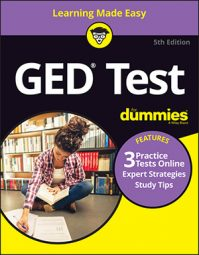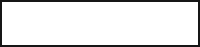##### GED Test 2022 / 2023 For Dummies with Online PracticeSome questions on the GED Math test will involve ratios. You use a ratio to compare the relative size of two numbers, by showing how many times the second number goes into the first (for example, 2 apples to 1 pear gives you a 2:1 ratio).

In the following practice questions, you have to find the ratio of the largest to the smallest departmental budget in a company, and then use ratios to convert a drawing to a wall-size mural.

## Practice questions

Use the following information to answer the first practice question.

In a large company, the top four positions are organized as follows:

Each department has the following budget:

1. What is the ratio of the largest budget to the smallest budget?2. Elena wants to draw a mural on the wall of her house. The wall is 9 feet high and 17 feet long. To plan the mural, she draws a scale drawing of the area for the mural on a piece of paper 11 inches long. How high, in inches, should the drawing be to maintain scale?

A. 6.2 B. 5.8 C. 8.5 D. 9.0

1. The correct answer is 7:1.

This question tests your data-analysis skills by asking you to interpret a chart and answer a question involving calculation.

The largest budget is the Operations budget, while the smallest budget is Human Resources. The ratio between these two budgets is 14.7 to 2.1 or 7:1 (dividing both sides by 2.1).

If you wanted to do this in your head, notice that 14:2 (the approximate ratio between the Operations budget and the Human Resources budget) is double 7:1.

2. The correct answer is Choice (B). This problem tests your skills in geometry and involves similarity of geometrical figures. To draw a scale drawing, the lengths and widths must be reduced in the same ratio. If the wall is 17 feet or

and the paper is 11 inches long, the ratio of paper length to real length is 11:204. The width of the drawing must stay in the same ratio. If the height of the drawing is H, then

In other words,

By cross-multiplying, you get

Note that the answer is rounded.